Fibonacci retracement levels are horizontal lines on a trading chart that indicate where possible future price support and price resistance levels are likely to occur. Fibonacci retracement levels exist only between past low and past high price zones. But can we draw Fibonacci levels above the previous high and below the previous low?

## What are Fibonacci Expansion Levels?

Fibonacci Expansion Levels or Fibonacci extension levels are technical analysis lines, calculated as the percentage between high and low prices, which can be drawn above the highest or below the lowest low price level. The most common Fibonacci Expansion levels are 161.8%, 200%, and 261.8%. Fibonacci projection levels are the second name for Fibonacci expansion levels because price levels go beyond 100%.

Fibonacci Expansion Levels or Fibonacci Extension levels follow the same logic as Fibonacci retracements. Metatrader platform has fib extension and fib retracement (standard) indicators, and it draws automatic lines on the chart after the user picks high and low levels.
The most common levels used for Fibonacci retracements are 23.6%, 38.2%, 61.8%.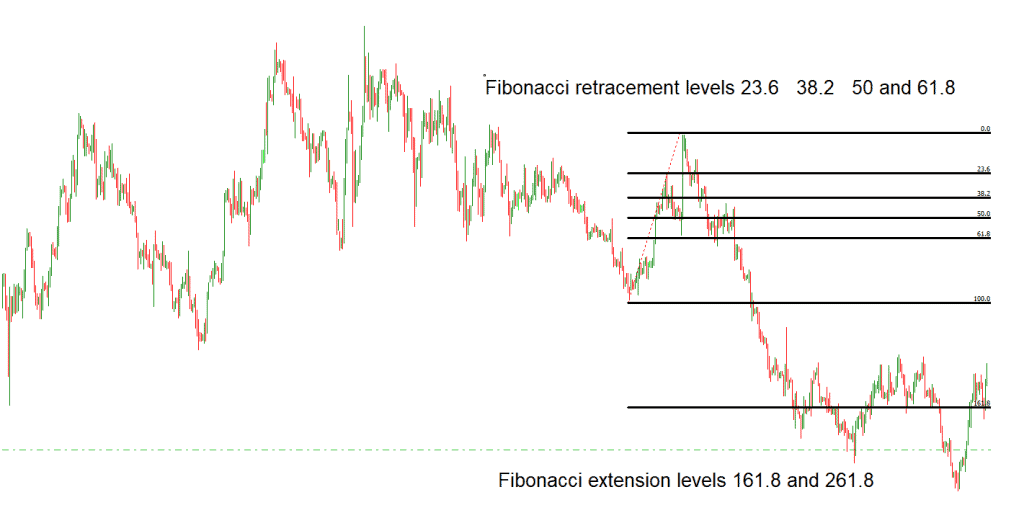Fibonacci extension levels facts:

• Fibonacci ratios are derived from the Fibonacci sequence and are seen universally in varied natural elements.
• Fibonacci extension levels show the extension of a trend move.
• Fibonacci extension levels are 161.8%, 200%, and 261.8%, etc.
• Fibonacci extension levels show how far the next price above 100% wave could move.
• Extension levels are just important hypothetical levels; there is no guarantee that price will react to them.

Let us see what the best Fibonacci expansion levels are:

## What are Important Fibonacci extension levels?

For traders, the most important Fibonacci extension levels are 161.8%, 200%, and 261.8%. These three levels can help traders to determine target levels in a bull or bear market when the price is very close to the highest high or lowest low. In that case, important Fibonacci expansion levels are the best for predicting the next high (bullish trend) or the next low (bearish trend).

Auto Fibonacci Extension Indicator MT4 represents an indicator that draws retracement levels and expansion levels in an automated way on the MT4. Below you can download the Fibo levels indicator:

In this indicator are added Fibonacci expansion levels using code:

double level_array={0,0.236,0.382,0.5,0.618,0.764,1,1.618,2.618,4.236};
string leveldesc_array={“0″,”23.6%”,”38.2%”,”50%”,”61.8%”,”76.4%”,”100%”,”161.8%”,”261.80%”,”423.6%”};
int level_count;
string level_name;

The indicator will automatically draw only levels seen on the chart. If the price level is too far, it will not be visible. For example, we see only 161.8% expansion Fib in the chart below. Level 261.8% and 423.6% are not visible because they are too far.If you need an automated indicator that will draw Fibonacci levels automatic based on the latest number of periods, then you can download it below:

Technical traders rely extensively on the Fibonacci Retracement tool to scour the market for opportunities and rake in gains from the trading market. This tool is easy to use as its foundation is based on the Fibonacci number sequence like 0,1,1,2,3,5,8, etc. Once you divide these Fibonacci numbers with adjacent numbers, you will acquire a Fibonacci ratio to further help you in the technical trading strategy.

The first step to plotting this indicator in the chart is to locate the high and low points, which often pose some errors for traders. You can also encounter blunders when using this indicator, but once you become more proficient, using it will be a breeze. However, if you want to speed things up, Auto Fibonacci Retracement tool is a viable choice, which takes away the burden of finding the highs and lows and does the plotting for you. The most versatile quality of the Auto Fibonacci Indicator is that you can use it in different time frames and currency pairs.

## How to use the Auto Fibonacci Retracement Indicator?

To use Auto Fibonacci Retracement indicator, you just need to drag and drop the indicator in the MT4 platform and mark wished lowest and highest price levels on the chart. Then indicator will mark all retracement lines between high and low prices on the chart, and then expansion levels above and below chosen high and low prices.

The levels in this indicator range from 0-100%. If the market at the current time is in a bearish condition, you will notice the 0 point is at the peak while the 100 lays below, and the opposite happens when there is a bullish condition in the market. Once the market jumps to a new high, the market will retrace different Fibonacci Retracement Levels.

To comprehend the above part, let’s take an example of an uptrend where it retraces up to the 38.2% Fibonacci level. Now you will monitor the market until it returns to the 38.2% level and then takes a buy position in the market. Then, your stop-loss point will come below the 50% Fibonacci level. Finally, the take profit point can be established at the following high resistance level to know the ideal exit points.

This price will also go up to the 50% level in some instances. While the traders highly praise the level of 61.8% as it gives excellent pullback opportunities. You can also trade on the 78.6% level on the Fibonacci Retracement tool, as it makes up for a good reversal point, but traders often pay no attention to it.

You can also utilize the Fibonacci Retracement levels for take-profit and continue with the trend wave. For instance, if the uptrend in the market is retracing, you can acquire a short position with a profit point of 68.1%.

If you observe a chart depicting the price motion of the U.S. dollar in opposition to the Japanese Yen in the timeframe of half-hour, the retracement point goes beyond the level of 38.6% and 50%. You will witness a pullback from the level of 61.8% to 38.6% before continuing the bullish trend in the market.

Based on your technical strategy, you will enter the trading market with your stop loss point above the most recent high swing. If you want to exit a trade, do so at the 78.6% or 100% Fibonacci level, which collides with the following support zone. Traders also utilize the Fibonacci expansion tool to pick up profits from the market.

This Auto Fibonacci Retracement Tool cuts off the time taking process of plotting and makes it easier to trade with its automated functions. However, the level is not an indicator of the exit and entry signals, but the Fibonacci levels are. Hence, just like any other technical trading tool, using this indicator with a blend of different tools would be a prudent choice.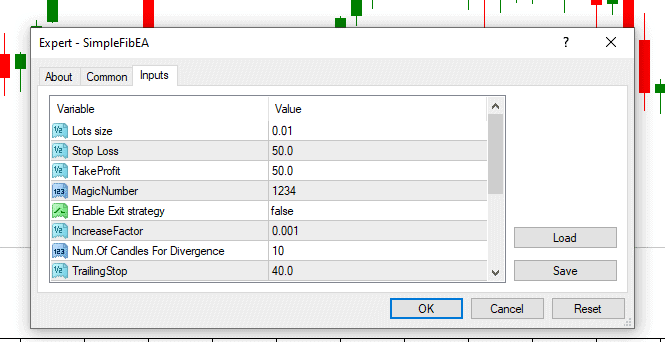## Main Facts about Fibonacci Expansion (Extension) levels and Fibonacci retracements

When it comes to technical analysis, the term that refers to any area of resistance or support is known as a Fibonacci retracement. It will also use a horizontal line to point where there might be resistance or support, and each of those levels will be labeled using percentages. That percentage amount is a pricing amount that has moved and been retraced. The levels within Fibonacci retracements are 78.6%, 61.8%, 38.2%, and 23.6%, while even though it may not be official, 50% can be used.

Fibonacci extension levels
The Fibonacci extensions show how far the next price wave could move above 100%. Common Fibonacci extension levels are 161.8%, 200%, 261.8% and 423.6%.

This is considered to be beneficial due to it being able to show highs and lows between different price points, and this creates the known level between the selected points.

For instance, if the price started at \$10 and suddenly dropped to \$3.82, it has done a retrace, and this percentage is a retraced 38.2%, a number of the Fibonacci retracement. These particular sets of numbers can be found everywhere in nature, so it is believed that these specific numbers are relevant within the financial market.

Formula Used to Find the Fibonacci Expansion (Extension) and Retracement Levels

An indicator will not have a formula, so when a trader applies an indicator to charts and picks two points, the lines drawn will show the moving percentage once selected.

For instance, if a price goes from \$15 to \$20, and the two prices have been selected to find the indicator, multiply the amount difference between the two prices by the Fibonacci Retracement percentages to find the levels. Therefore, you would take \$20 – (\$5 X 0.5) = \$17.50 and so on.

If a trader wanted to calculate the levels, you would follow the formula listed above and use the percentage of a particular price range that has been selected.
However, many ask where these particular sets of numbers originate from. They are all based on what is known as the Golden Ratio.
If a person created a specific number sequence that began with the number zero, then added one, and eventually continuously added the first two numbers, this string would continue indefinitely. For instance, it would look something like this:

0+1 = 1, 1+1=2, 1+2 = 3, 3+2 = 5, 5+3=8, 8+5 =13, 13+8=21, 21+13 = 34, 34+21=55, 55+34=89, and it would continue on just like this. The sums would make up the entire string of numbers and go on indefinitely.

All of the levels are going to come from this series of numbers. However, this excludes the first very few numbers, so when the series is going, if the number is divided by the number that comes next, you will end up with the level numbers. However, taking time to explore these options carefully, a person would notice that all of these percentages, except for 50%, will be considered an actual Fibonacci number as 50% is not seen as an official percentage. These percentages will be based on math calculations based on this particular number string.

Another exciting thing about the Golden Ratio is that 1.618 or 0.618 can be located within galaxy formations, historical architecture, artifacts, shells, and even sunflowers.

The Fibonacci retracements can determine stop-loss levels, help set target prices, and place entry orders. If a trader notices that a stock is moving up and after it moves, it ends up with a 61.8% retrace, then it begins to bounce once again. Because the bounce was considered at a level on the Fibonacci scale, and if the trend stays for a long time, a trader may decide to buy. A trader could create a stop loss within the 78.6% or 100% where it originally started.

These levels can also be utilized in various types of technical analysis. For instance, they are seen in the Elliott Wave theory and Gartley patterns. After a price has moved down or up significantly, it always seems to do when a price starts to retrace its path; these analyses will find the retrace and notice that it reverses to certain levels.
Unlike a moving average, these levels are often at a fixed price that will not usually change. A static nature will be needed to efficiently and quickly identify price levels. This allows investors and traders to react and anticipate when a level may be tested. The levels will often be an inflection point, indicating where a price action could be expected, whether a break or a rejection.

## How to use the Fibonacci extension on MT4?

To use the Fibonacci extension on MT4, choose Insert/Fibonacci/Expansion tool and select the highest high and lowest low price level on the chart. At that moment,  Fib levels will be added to the chart (see image below):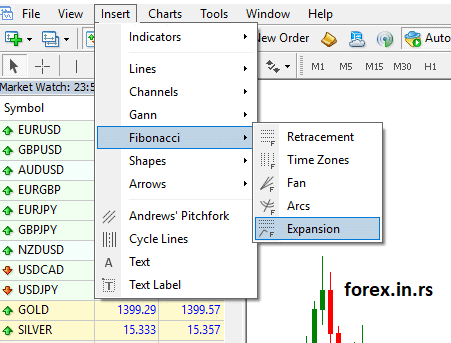If you pick Fib, levels retracements, or Expansion levels, you will get, in both cases, expansion levels. However, the expansion levels tool will give you only the two most common levels for your target.

How to trade with Fibonacci retracements and extensions?

After drawing Fib levels on the chart (retracements and extensions), we need to define the entry and exit strategy. Fib. Levels we can use as exit and entry levels. Example :

Step 1: Pick low and high on the chart.
Step 2: If the close hourly price is below 61.8% Fibonacci retracement, make a SELL trade.
Step 3: Put stop loss on High Price.
Step 4: Set your target to be either 161.8% expansion level or 200% expansion level, or 268.1% expansion level.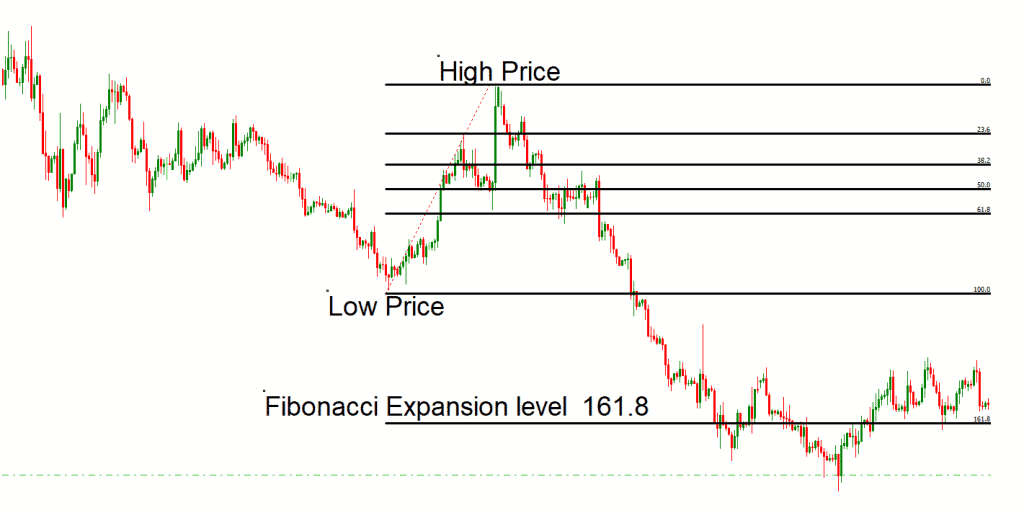You can cut a small number of lots on each target and move stop loss, whatever you like.

See this strategy for sell trade on the image below: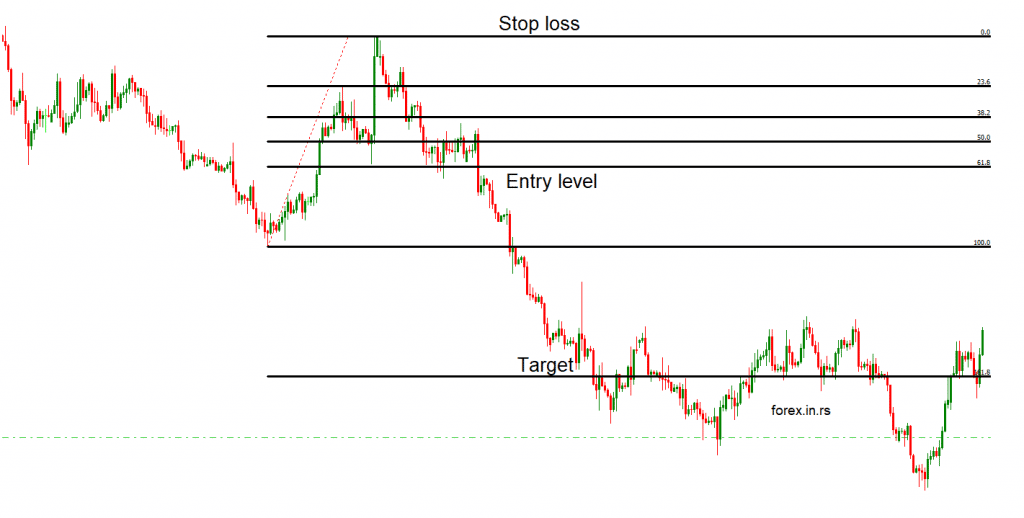## Differences Between Expansions (Extensions) and Retracements levels

The Fibonacci extensions are the percentages that go back to a trending direction, while the retracements are the percentages that pull back. So, for instance, if you have a stock that increases from \$5 up to \$10, but then it goes back down to \$6.75, then it is considered to be a retracement, but if that price happens to increase up to \$16, then it is called an extension.

Level Limitations
Even though retracement levels can show where a price may potentially find resistance or support, there are not any assurances that this special price will stop at that point. This can be seen as why a trader should look for other signals that may be utilized, such as prices bouncing off a particular level.

Another argument against a retracement level is that, due to several levels, the price could reverse near one of these levels, which can happen much more often than it would not. The overall problem is that a trader could struggle to locate which one would be helpful during any retracement that may be happening and analyzed.

The Key Takeaways
An indicator connects two points that a trader will view as relevant, often a low and high. Once an indicator has been selected and drawn on the chart, these levels will be considered fixed and will not change. The provided percentages will be locations where pricing may reverse or stall. Therefore, a trader should not solely rely on the levels. For instance, assuming that a price will reverse after hitting a particular Fibonacci level is detrimental. It could, but then it may not.

A Fibonacci retracement level can show areas of interest that may offer potential. For example, if traders are looking to buy, they will watch if the price stalls at a Fibonacci level and wait for it to bounce off that particular level before deciding to buy.
The most commonly used ratios will include 78.6%, 61.8%, 38.2%, and 23.6%, while 50% is seen as an unofficial retracement level. These percentages will show how much of a previous move was made, whether retracted or corrected.

The support and resistance levels determination is the most essential part of technical analysis. Therefore, the pivot points are a significant indicator of the price trends in the market.  One helpful indicator that helps determine the stop loss and take profit point is the Fibonacci Pivots Indicator.

Latest posts by Fxigor (see all)

Fxigor

Igor has been a trader since 2007. Currently, Igor works for several prop trading companies. He is an expert in financial niche, long-term trading, and weekly technical levels. The primary field of Igor's research is the application of machine learning in algorithmic trading. Education: Computer Engineering and Ph.D. in machine learning. Igor regularly publishes trading-related videos on the Fxigor Youtube channel. To contact Igor write on: igor@forex.in.rs

Trade gold and silver. Visit the broker's page and start trading high liquidity spot metals - the most traded instruments in the world.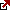Diversify your savings with a gold IRA.

Gold & silver portfolio-building assistance from product professionals.

If you want to trade stocks try: